Scan QR code or get instant email to install app

Question:

# 1212Which answer choice best provides the critical information regarding the following rational function: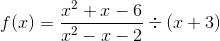A 1212Domain: x \u2260 -1, x \u2260 -3, x \u2260 2
Range: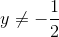,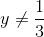, y \u2260 0
Horizontal Asymptote: y = 0
Vertical Asymptote: x = -1
y-intercept: (0, 1) End Behavior: f(x) approaches negative infinity as x approaches -1 from the left. f(x) approaches positive infinity as x approaches -1 from the right.
explanation

1212Begin by factoring the first expression and rewriting the division as an inverse multiplication: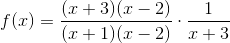The function shows a discontinuity at x = 2 and x = -3. It shows a vertical asymptote at x = -1.
To find the excluded range values corresponding to the discontinuities, substitute the x values into the reduced function: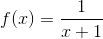. So: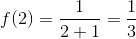and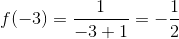Solving the function for x enables another excluded range value to be found: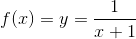, which gives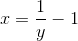, so y \u2260 0
From the reduced function:, it can be seen that as x increases, f(x) approaches 0, and that as x approaches -1 from the right, f(x) approaches infinity. As x decreases, f(x) approaches 0, and as x approaches -1 from the left, f(x) approaches negative infinity.
Lastly, the y intercept: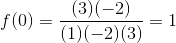, giving the point (0, 1)